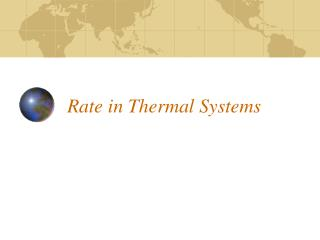# Rate in Thermal Systems - PowerPoint PPT PresentationDownload PresentationRate in Thermal Systems

Rate in Thermal SystemsDownload Presentation## Rate in Thermal Systems

- - - - - - - - - - - - - - - - - - - - - - - - - - - E N D - - - - - - - - - - - - - - - - - - - - - - - - - - -
##### Presentation Transcript

1. Rate in Thermal Systems

2. Objectives • Define Heat flow rate and its SI and English units of measure. • Describe the heat transfer processes of conduction, convection and radiation. • Solve heat transfer rate problems using the heat conduction equation.

3. Basics of heat flow • Always flows from regions of higher temperature to regions of lower temperature. • Always caused by a difference of temperature. • Heat is the exciting of molecules.

4. Rate Review How much Rate = Time Distance miles Mechanical Rate = = Time hour Volume or mass gallons Fluid Rate = = Time minute coulombs Charge Electrical Rate = = Time second

5. Heat Flow Rate heat transferred Thermal Rate= Time calories (cal) • SI Units Q joules (J) Q =  t British Thermal Units (BTU) English Units Foot-pounds(ft•lb) seconds (s), minutes (min) or hours (h)

6. Heat Conduction • The process of transferring heat from one molecule to another. • The collision of vibrating molecules. • The transfer of thermal energy arising form a temperature difference between adjacent parts of a body.

7. Thermal Conductivity (k) • The measure of a material’s ability to conduct heat. • Metals have a large thermal conductivity because they are good heat conductors • Wood has low thermal conductivity because it is a poor heat conductor.

8. Heat Conduction Rate thermal conductivity cross-sectional area temperature difference x x Heat Conduction = Rate thickness (k) x (A) x (t) • (-) Q = (x)

9. Convection • Transfer of heat by movement of a fluid. Natural convection - earths atmosphere Low Temperature Warm Fluid rises Cool fluid sinks Ponds and lakes “turning over” Forced convection – fan or pump is used High Temperature Human circulatory system

10. Radiation • Transfer of energy by electromagnetic waves. • All objects radiate electromagnetic energy. • The energy radiated by an object depends on the objects temperature, surface area, and material composition of the surface. • Radiation transfers energy from one body to another through empty space – it does not use a medium. Energy from the sun to all the planets is done through radiation.

11. Summary • Heat flow rate is the amount of thermal energy transferred per unit of time. • Heat is transferred from a high temperature object or region to a low temperature object or region. • Heat conduction is the transfer of thermal energy within an object due to a temperature difference between adjacent regions of the object. • The thermal conductivity of a material is a measure of its ability to conduct heat. • Convection is a transfer of heat by movement of fluid. Convection can be natural or forced. • Radiation is a transfer of energy by electromagnetic waves.